Prealgebra 2e

# 10.3Multiply Polynomials

Prealgebra 2e10.3 Multiply Polynomials

### Learning Objectives

By the end of this section, you will be able to:

• Multiply a polynomial by a monomial
• Multiply a binomial by a binomial
• Multiply a trinomial by a binomial

### Be Prepared 10.6

Before you get started, take this readiness quiz.

Distribute: $2(x+3).2(x+3).$
If you missed the problem, review Example 7.17.

### Be Prepared 10.7

Distribute: $−11(4−3a).−11(4−3a).$
If you missed the problem, review Example 7.26.

### Be Prepared 10.8

Combine like terms: $x2+9x+7x+63.x2+9x+7x+63.$
If you missed the problem, review Example 2.21.

### Multiply a Polynomial by a Monomial

In Distributive Property you learned to use the Distributive Property to simplify expressions such as $2(x−3).2(x−3).$ You multiplied both terms in the parentheses, $xand3,xand3,$ by $2,2,$ to get $2x−6.2x−6.$ With this chapter's new vocabulary, you can say you were multiplying a binomial, $x−3,x−3,$ by a monomial, $2.2.$ Multiplying a binomial by a monomial is nothing new for you!

### Example 10.28

Multiply: $3(x+7).3(x+7).$

### Try It 10.55

Multiply: $6(x+8).6(x+8).$

### Try It 10.56

Multiply: $2(y+12).2(y+12).$

### Example 10.29

Multiply: $x(x−8).x(x−8).$

### Try It 10.57

Multiply: $y(y−9).y(y−9).$

### Try It 10.58

Multiply: $p(p−13).p(p−13).$

### Example 10.30

Multiply: $10x(4x+y).10x(4x+y).$

### Try It 10.59

Multiply: $8x(x+3y).8x(x+3y).$

### Try It 10.60

Multiply: $3r(6r+s).3r(6r+s).$

Multiplying a monomial by a trinomial works in much the same way.

### Example 10.31

Multiply: $−2x(5x2+7x−3).−2x(5x2+7x−3).$

### Try It 10.61

Multiply: $−4y(8y2+5y−9).−4y(8y2+5y−9).$

### Try It 10.62

Multiply: $−6x(9x2+x−1).−6x(9x2+x−1).$

### Example 10.32

Multiply: $4y3(y2−8y+1).4y3(y2−8y+1).$

### Try It 10.63

Multiply: $3x2(4x2−3x+9).3x2(4x2−3x+9).$

### Try It 10.64

Multiply: $8y2(3y2−2y−4).8y2(3y2−2y−4).$

Now we will have the monomial as the second factor.

### Example 10.33

Multiply: $(x+3)p.(x+3)p.$

### Try It 10.65

Multiply: $(x+8)p.(x+8)p.$

### Try It 10.66

Multiply: $(a+4)p.(a+4)p.$

### Multiply a Binomial by a Binomial

Just like there are different ways to represent multiplication of numbers, there are several methods that can be used to multiply a binomial times a binomial.

#### Using the Distributive Property

We will start by using the Distributive Property. Look again at Example 10.33.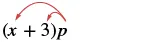We distributed the $pp$ to get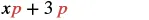What if we have $(x+7)(x+7)$ instead of $pp$?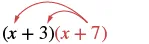Distribute $(x+7)(x+7)$.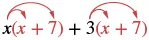Distribute again. $x2+7x+3x+21x2+7x+3x+21$ Combine like terms. $x2+10x+21x2+10x+21$

Notice that before combining like terms, we had four terms. We multiplied the two terms of the first binomial by the two terms of the second binomial—four multiplications.

Be careful to distinguish between a sum and a product.

$SumProductx+xx·x2xx2combine like termsadd exponents of like basesSumProductx+xx·x2xx2combine like termsadd exponents of like bases$

### Example 10.34

Multiply: $(x+6)(x+8).(x+6)(x+8).$

### Try It 10.67

Multiply: $(x+8)(x+9).(x+8)(x+9).$

### Try It 10.68

Multiply: $(a+4)(a+5).(a+4)(a+5).$

Now we'll see how to multiply binomials where the variable has a coefficient.

### Example 10.35

Multiply: $(2x+9)(3x+4).(2x+9)(3x+4).$

### Try It 10.69

Multiply: $(5x+9)(4x+3).(5x+9)(4x+3).$

### Try It 10.70

Multiply: $(10m+9)(8m+7).(10m+9)(8m+7).$

In the previous examples, the binomials were sums. When there are differences, we pay special attention to make sure the signs of the product are correct.

### Example 10.36

Multiply: $(4y+3)(6y−5).(4y+3)(6y−5).$

### Try It 10.71

Multiply: $(7y+1)(8y−3).(7y+1)(8y−3).$

### Try It 10.72

Multiply: $(3x+2)(5x−8).(3x+2)(5x−8).$

Up to this point, the product of two binomials has been a trinomial. This is not always the case.

### Example 10.37

Multiply: $(x+2)(x−y).(x+2)(x−y).$

### Try It 10.73

Multiply: $(x+5)(x−y).(x+5)(x−y).$

### Try It 10.74

Multiply: $(x+2y)(x−1).(x+2y)(x−1).$

#### Using the FOIL Method

Remember that when you multiply a binomial by a binomial you get four terms. Sometimes you can combine like terms to get a trinomial, but sometimes there are no like terms to combine. Let's look at the last example again and pay particular attention to how we got the four terms.

$(x+2)(x−y)(x+2)(x−y)$
$x2−xy+2x−2yx2−xy+2x−2y$

Where did the first term, $x2,x2,$ come from?

It is the product of $xandx,xandx,$ the first terms in $(x+2)and(x−y).(x+2)and(x−y).$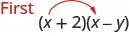The next term, $−xy,−xy,$ is the product of $xand−y,xand−y,$ the two outer terms.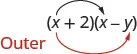The third term, $+2x,+2x,$ is the product of $2andx,2andx,$ the two inner terms.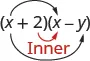And the last term, $−2y,−2y,$ came from multiplying the two last terms.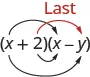We abbreviate “First, Outer, Inner, Last” as FOIL. The letters stand for ‘First, Outer, Inner, Last’. The word FOIL is easy to remember and ensures we find all four products. We might say we use the FOIL method to multiply two binomials.Let's look at $(x+3)(x+7)(x+3)(x+7)$ again. Now we will work through an example where we use the FOIL pattern to multiply two binomials.

### Example 10.38

Multiply using the FOIL method: $(x+6)(x+9).(x+6)(x+9).$

### Try It 10.75

Multiply using the FOIL method: $(x+7)(x+8).(x+7)(x+8).$

### Try It 10.76

Multiply using the FOIL method: $(y+14)(y+2).(y+14)(y+2).$

We summarize the steps of the FOIL method below. The FOIL method only applies to multiplying binomials, not other polynomials!

### How To

#### Use the FOIL method for multiplying two binomials.

1. Step 1. Multiply the First terms.
2. Step 2. Multiply the Outer terms.
3. Step 3. Multiply the Inner terms.
4. Step 4. Multiply the Last terms.
5. Step 5. Combine like terms, when possible.### Example 10.39

Multiply: $(y−8)(y+6).(y−8)(y+6).$

### Try It 10.77

Multiply: $(y−3)(y+8).(y−3)(y+8).$

### Try It 10.78

Multiply: $(q−4)(q+5).(q−4)(q+5).$

### Example 10.40

Multiply: $(2a+3)(3a−1).(2a+3)(3a−1).$

### Try It 10.79

Multiply: $(4a+9)(5a−2).(4a+9)(5a−2).$

### Try It 10.80

Multiply: $(7x+4)(7x−8).(7x+4)(7x−8).$

### Example 10.41

Multiply: $(5x−y)(2x−7).(5x−y)(2x−7).$

### Try It 10.81

Multiply: $(12x−y)(x−5).(12x−y)(x−5).$

### Try It 10.82

Multiply: $(6a−b)(2a−9).(6a−b)(2a−9).$

#### Using the Vertical Method

The FOIL method is usually the quickest method for multiplying two binomials, but it works only for binomials. You can use the Distributive Property to find the product of any two polynomials. Another method that works for all polynomials is the Vertical Method. It is very much like the method you use to multiply whole numbers. Look carefully at this example of multiplying two-digit numbers.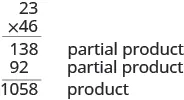You start by multiplying $2323$ by $66$ to get $138.138.$

Then you multiply $2323$ by $4,4,$ lining up the partial product in the correct columns.

Last, you add the partial products.

Now we'll apply this same method to multiply two binomials.

### Example 10.42

Multiply using the vertical method: $(5x−1)(2x−7).(5x−1)(2x−7).$

### Try It 10.83

Multiply using the vertical method: $(4m−9)(3m−7).(4m−9)(3m−7).$

### Try It 10.84

Multiply using the vertical method: $(6n−5)(7n−2).(6n−5)(7n−2).$

We have now used three methods for multiplying binomials. Be sure to practice each method, and try to decide which one you prefer. The three methods are listed here to help you remember them.

### Multiplying Two Binomials

To multiply binomials, use the:
• Distributive Property
• FOIL Method
• Vertical Method
Remember, FOIL only works when multiplying two binomials.

### Multiply a Trinomial by a Binomial

We have multiplied monomials by monomials, monomials by polynomials, and binomials by binomials. Now we're ready to multiply a trinomial by a binomial. Remember, the FOIL method will not work in this case, but we can use either the Distributive Property or the Vertical Method. We first look at an example using the Distributive Property.

### Example 10.43

Multiply using the Distributive Property: $(x+3)(2x2−5x+8).(x+3)(2x2−5x+8).$

### Try It 10.85

Multiply using the Distributive Property: $(y−1)(y2−7y+2).(y−1)(y2−7y+2).$

### Try It 10.86

Multiply using the Distributive Property: $(x+2)(3x2−4x+5).(x+2)(3x2−4x+5).$

Now let's do this same multiplication using the Vertical Method.

### Example 10.44

Multiply using the Vertical Method: $(x+3)(2x2−5x+8).(x+3)(2x2−5x+8).$

### Try It 10.87

Multiply using the Vertical Method: $(y−1)(y2−7y+2).(y−1)(y2−7y+2).$

### Try It 10.88

Multiply using the Vertical Method: $(x+2)(3x2−4x+5).(x+2)(3x2−4x+5).$

### Section 10.3 Exercises

#### Practice Makes Perfect

Multiply a Polynomial by a Monomial

In the following exercises, multiply.

145.

$4 ( x + 10 ) 4 ( x + 10 )$

146.

$6 ( y + 8 ) 6 ( y + 8 )$

147.

$15 ( r − 24 ) 15 ( r − 24 )$

148.

$12 ( v − 30 ) 12 ( v − 30 )$

149.

$−3 ( m + 11 ) −3 ( m + 11 )$

150.

$−4 ( p + 15 ) −4 ( p + 15 )$

151.

$−8 ( z − 5 ) −8 ( z − 5 )$

152.

$−3 ( x − 9 ) −3 ( x − 9 )$

153.

$u ( u + 5 ) u ( u + 5 )$

154.

$q ( q + 7 ) q ( q + 7 )$

155.

$n ( n 2 − 3 n ) n ( n 2 − 3 n )$

156.

$s ( s 2 − 6 s ) s ( s 2 − 6 s )$

157.

$12 x ( x − 10 ) 12 x ( x − 10 )$

158.

$9 m ( m − 11 ) 9 m ( m − 11 )$

159.

$−9 a ( 3 a + 5 ) −9 a ( 3 a + 5 )$

160.

$−4 p ( 2 p + 7 ) −4 p ( 2 p + 7 )$

161.

$6 x ( 4 x + y ) 6 x ( 4 x + y )$

162.

$5 a ( 9 a + b ) 5 a ( 9 a + b )$

163.

$5 p ( 11 p − 5 q ) 5 p ( 11 p − 5 q )$

164.

$12 u ( 3 u − 4 v ) 12 u ( 3 u − 4 v )$

165.

$3 ( v 2 + 10 v + 25 ) 3 ( v 2 + 10 v + 25 )$

166.

$6 ( x 2 + 8 x + 16 ) 6 ( x 2 + 8 x + 16 )$

167.

$2 n ( 4 n 2 − 4 n + 1 ) 2 n ( 4 n 2 − 4 n + 1 )$

168.

$3 r ( 2 r 2 − 6 r + 2 ) 3 r ( 2 r 2 − 6 r + 2 )$

169.

$−8 y ( y 2 + 2 y − 15 ) −8 y ( y 2 + 2 y − 15 )$

170.

$−5 m ( m 2 + 3 m − 18 ) −5 m ( m 2 + 3 m − 18 )$

171.

$5 q 3 ( q 2 − 2 q + 6 ) 5 q 3 ( q 2 − 2 q + 6 )$

172.

$9 r 3 ( r 2 − 3 r + 5 ) 9 r 3 ( r 2 − 3 r + 5 )$

173.

$−4 z 2 ( 3 z 2 + 12 z − 1 ) −4 z 2 ( 3 z 2 + 12 z − 1 )$

174.

$−3 x 2 ( 7 x 2 + 10 x − 1 ) −3 x 2 ( 7 x 2 + 10 x − 1 )$

175.

$( 2 y − 9 ) y ( 2 y − 9 ) y$

176.

$( 8 b − 1 ) b ( 8 b − 1 ) b$

177.

$( w − 6 ) · 8 ( w − 6 ) · 8$

178.

$( k − 4 ) · 5 ( k − 4 ) · 5$

Multiply a Binomial by a Binomial

In the following exercises, multiply the following binomials using: the Distributive Property the FOIL method the Vertical method

179.

$( x + 4 ) ( x + 6 ) ( x + 4 ) ( x + 6 )$

180.

$( u + 8 ) ( u + 2 ) ( u + 8 ) ( u + 2 )$

181.

$( n + 12 ) ( n − 3 ) ( n + 12 ) ( n − 3 )$

182.

$( y + 3 ) ( y − 9 ) ( y + 3 ) ( y − 9 )$

In the following exercises, multiply the following binomials. Use any method.

183.

$( y + 8 ) ( y + 3 ) ( y + 8 ) ( y + 3 )$

184.

$( x + 5 ) ( x + 9 ) ( x + 5 ) ( x + 9 )$

185.

$( a + 6 ) ( a + 16 ) ( a + 6 ) ( a + 16 )$

186.

$( q + 8 ) ( q + 12 ) ( q + 8 ) ( q + 12 )$

187.

$( u − 5 ) ( u − 9 ) ( u − 5 ) ( u − 9 )$

188.

$( r − 6 ) ( r − 2 ) ( r − 6 ) ( r − 2 )$

189.

$( z − 10 ) ( z − 22 ) ( z − 10 ) ( z − 22 )$

190.

$( b − 5 ) ( b − 24 ) ( b − 5 ) ( b − 24 )$

191.

$( x − 4 ) ( x + 7 ) ( x − 4 ) ( x + 7 )$

192.

$( s − 3 ) ( s + 8 ) ( s − 3 ) ( s + 8 )$

193.

$( v + 12 ) ( v − 5 ) ( v + 12 ) ( v − 5 )$

194.

$( d + 15 ) ( d − 4 ) ( d + 15 ) ( d − 4 )$

195.

$( 6 n + 5 ) ( n + 1 ) ( 6 n + 5 ) ( n + 1 )$

196.

$( 7 y + 1 ) ( y + 3 ) ( 7 y + 1 ) ( y + 3 )$

197.

$( 2 m − 9 ) ( 10 m + 1 ) ( 2 m − 9 ) ( 10 m + 1 )$

198.

$( 5 r − 4 ) ( 12 r + 1 ) ( 5 r − 4 ) ( 12 r + 1 )$

199.

$( 4 c − 1 ) ( 4 c + 1 ) ( 4 c − 1 ) ( 4 c + 1 )$

200.

$( 8 n − 1 ) ( 8 n + 1 ) ( 8 n − 1 ) ( 8 n + 1 )$

201.

$( 3 u − 8 ) ( 5 u − 14 ) ( 3 u − 8 ) ( 5 u − 14 )$

202.

$( 2 q − 5 ) ( 7 q − 11 ) ( 2 q − 5 ) ( 7 q − 11 )$

203.

$( a + b ) ( 2 a + 3 b ) ( a + b ) ( 2 a + 3 b )$

204.

$( r + s ) ( 3 r + 2 s ) ( r + s ) ( 3 r + 2 s )$

205.

$( 5 x − y ) ( x − 4 ) ( 5 x − y ) ( x − 4 )$

206.

$( 4 z − y ) ( z − 6 ) ( 4 z − y ) ( z − 6 )$

Multiply a Trinomial by a Binomial

In the following exercises, multiply using the Distributive Property and the Vertical Method.

207.

$( u + 4 ) ( u 2 + 3 u + 2 ) ( u + 4 ) ( u 2 + 3 u + 2 )$

208.

$( x + 5 ) ( x 2 + 8 x + 3 ) ( x + 5 ) ( x 2 + 8 x + 3 )$

209.

$( a + 10 ) ( 3 a 2 + a − 5 ) ( a + 10 ) ( 3 a 2 + a − 5 )$

210.

$( n + 8 ) ( 4 n 2 + n − 7 ) ( n + 8 ) ( 4 n 2 + n − 7 )$

In the following exercises, multiply. Use either method.

211.

$( y − 6 ) ( y 2 − 10 y + 9 ) ( y − 6 ) ( y 2 − 10 y + 9 )$

212.

$( k − 3 ) ( k 2 − 8 k + 7 ) ( k − 3 ) ( k 2 − 8 k + 7 )$

213.

$( 2 x + 1 ) ( x 2 − 5 x − 6 ) ( 2 x + 1 ) ( x 2 − 5 x − 6 )$

214.

$( 5 v + 1 ) ( v 2 − 6 v − 10 ) ( 5 v + 1 ) ( v 2 − 6 v − 10 )$

#### Everyday Math

215.

Mental math You can use binomial multiplication to multiply numbers without a calculator. Say you need to multiply $1313$ times $15.15.$ Think of $1313$ as $10+310+3$ and $1515$ as $10+5.10+5.$

1. Multiply $(10+3)(10+5)(10+3)(10+5)$ by the FOIL method.
2. Multiply $13·1513·15$ without using a calculator.
3. Which way is easier for you? Why?
216.

Mental math You can use binomial multiplication to multiply numbers without a calculator. Say you need to multiply $1818$ times $17.17.$ Think of $1818$ as $20−220−2$ and $1717$ as $20−3.20−3.$

1. Multiply $(20−2)(20−3)(20−2)(20−3)$ by the FOIL method.
2. Multiply $18·1718·17$ without using a calculator.
3. Which way is easier for you? Why?

#### Writing Exercises

217.

Which method do you prefer to use when multiplying two binomials—the Distributive Property, the FOIL method, or the Vertical Method? Why?

218.

Which method do you prefer to use when multiplying a trinomial by a binomial—the Distributive Property or the Vertical Method? Why?

#### Self Check

After completing the exercises, use this checklist to evaluate your mastery of the objectives of this section.

What does this checklist tell you about your mastery of this section? What steps will you take to improve?

Order a print copy

As an Amazon Associate we earn from qualifying purchases.